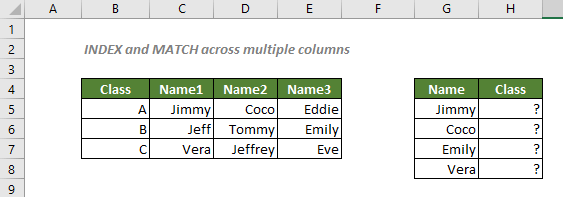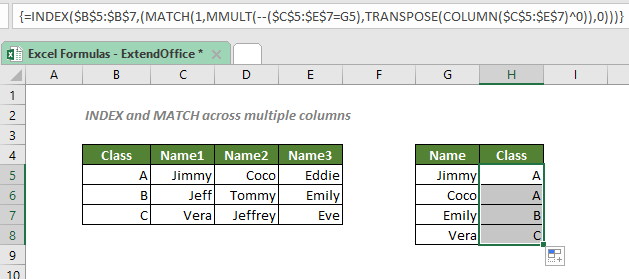Note: The other languages of the website are Google-translated. Back to English

## INDEX and MATCH across multiple columns

To lookup a value by matching across multiple columns, an array formula based on the INDEX and MATCH functions that incorporateswill do you a favor.#### How to lookup a value by matching across multiple columns?

To fill in the corresponding class of each student as the above table shown, where the information is listed across multiple columns, you can first use the trick of the MMULT, TRANSPOSE and COLUMN function to produce a matrix array. Then the MATCH function will give you the position of your lookup value, which will be fed to INDEX to retrieve the value you are looking for in the array.

#### Generic syntax

=INDEX(return_range,(MATCH(1,MMULT(--(lookup_array=lookup_value),TRANSPOSE(COLUMN(lookup_array)^0)),0)))

√ Note: This is an array formula that requires you to enter with Ctrl + Shift + Enter.

• return_range: The range where you want the formula to return the class information from. Here refers to the class range.
• lookup_value: The value the formula used to find its corresponding class information. Here refers to the given name.
• lookup_array: The range of cells where the lookup_value is listed; The range with the values to compare with the lookup_value. Here refers to the name range.
• match_type 0: Forces MATCH to find the first value that exactly equals to the lookup_value.

To find the class of Jimmy, please copy or enter the formula below in the cell H5, and press Ctrl + Shift + Enter to get the result:

=INDEX(\$B\$5:\$B\$7,(MATCH(1,MMULT(--(\$C\$5:\$E\$7=G5),TRANSPOSE(COLUMN(\$C\$5:\$E\$7)^0)),0)))

√ Note: The dollar signs (\$) above indicate absolute references, which means the name and class ranges in the formula won't change when you move or copy the formula to other cells. Note that you should not add dollar signs to the cell reference that represents the lookup value, since you want it to be relative when you copy it to other cells. After you enter the formula, drag the fill handle down to apply the formula to the below cells.#### Explanation of the formula

=INDEX(\$B\$5:\$B\$7,(MATCH(1,MMULT(--(\$C\$5:\$E\$7=G5),TRANSPOSE(COLUMN(\$C\$5:\$E\$7)^0)),0)))

• --(\$C\$5:\$E\$7=G5): This segment checks each value in the range \$C\$5:\$E\$7 if they equal to the value in the cell G5, and generates a TRUE and FALSE array like this:
{TRUE,FALSE,FALSE;FALSE,FALSE,FALSE;FALSE,FALSE,FALSE}.
The double negative will then convert the TRUEs and FALSEs to 1s and 0s to yield an array like this:
{1,0,0;0,0,0;0,0,0}.
• COLUMN(\$C\$5:\$E\$7): The COLUMN function returns the column numbers for the range \$C\$5:\$E\$7 in an array like this: {3,4,5}.
• TRANSPOSE(COLUMN(\$C\$5:\$E\$7)^0) = TRANSPOSE({3,4,5}^0): After raising the power to 0, all the numbers in the array {3,4,5} will be converted to 1: {1,1,1}. The TRANSPOSE function then converts the column array to a row array like this: {1;1;1}.
• MMULT(--(\$C\$5:\$E\$7=G5),TRANSPOSE(COLUMN(\$C\$5:\$E\$7)^0)) = MMULT({1,0,0;0,0,0;0,0,0},{1;1;1}): The MMULT function returns the matrix product of the two arrays like this: {1;0;0}.
• MATCH(1,MMULT(--(\$C\$5:\$E\$7=G5),TRANSPOSE(COLUMN(\$C\$5:\$E\$7)^0)),0) = MATCH(1,{1;0;0},0): The match_type 0 forces the MATCH function to return the position of the first match of 1 in the array {1;0;0}, which is 1.
• INDEX(\$B\$5:\$B\$7,(MATCH(1,MMULT(--(\$C\$5:\$E\$7=G5),TRANSPOSE(COLUMN(\$C\$5:\$E\$7)^0)),0))) = INDEX(\$B\$5:\$B\$7,1): The INDEX function returns the 1st value in the class range \$B\$5:\$B\$7, which is A.

To easily lookup a value by matching across multiple columns, you can also use our professional Excel add-in Kutools For Excel. See the instruction here to accomplish the mission.

#### Related functions

Excel INDEX function

The Excel INDEX function returns the displayed value based on a given position from a range or an array.

Excel MATCH function

The Excel MATCH function searches for a specific value in a range of cells, and returns the relative position of the value.

Excel MMULT function

The Excel MMULT function returns the matrix product of two arrays. The array result has the same number of rows as array1 and the same number of columns as array2.

Excel TRANSPOSE function

The Excel TRANSPOSE function rotates the orientation of a range or array. For example, it can rotate a table that arranged horizontally in rows to vertically in columns or vice versa.

Excel COLUMN function

The COLUMN function returns the number of column which formula appears or returns the column number of given reference. For example, formula =COLUMN(BD) returns 56.

#### Related Formulas

Multiple-criteria lookup with INDEX and MATCH

When dealing with a big database in an Excel spreadsheet with several columns and row captions, it’s always tricky to find something that meets multiple criteria. In this case, you can use an array formula with the INDEX and MATCH functions.

Two-way lookup with INDEX and MATCH

To search for something across both rows and columns in Excel, or we say to lookup a value at the intersection of the specific row and column, we can use the help of INDEX and MATCH functions.

Lookup closest match value with multiple criteria

In some cases, you may need to lookup the closest or approximate match value based on more than one criteria. With the combination of INDEX, MATCH and IF functions, you can quickly get it done in Excel.

### The Best Office Productivity Tools

#### Kutools for Excel - Helps You To Stand Out From Crowd

Would you like to complete your daily work quickly and perfectly? Kutools for Excel brings 300 powerful advanced features (Combine workbooks, sum by color, split cell contents, convert date, and so on...) and save 80% time for you.

• Designed for 1500 work scenarios, helps you solve 80% Excel problems.
• Reduce thousands of keyboard and mouse clicks every day, relieve your tired eyes and hands.
• Become an Excel expert in 3 minutes. No longer need to remember any painful formulas and VBA codes.
• 30-day unlimited free trial. 60-day money back guarantee. Free upgrade and support for 2 years.#### Office Tab - Enable Tabbed Reading and Editing in Microsoft Office (include Excel)

• One second to switch between dozens of open documents!
• Reduce hundreds of mouse clicks for you every day, say goodbye to mouse hand.
• Increases your productivity by 50% when viewing and editing multiple documents.
• Brings Efficient Tabs to Office (include Excel), Just Like Chrome, Firefox, And New Internet Explorer.